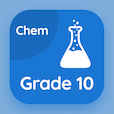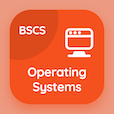Class 10 Online Courses

Grade 10 Chemistry MCQs PDF - Topics

# Reversible Reactions and Dynamic Equilibrium MCQ Quiz Online

Practice Reversible reactions and dynamic equilibrium Multiple Choice Questions (MCQ) to solve reversible reactions and dynamic equilibrium quiz answers PDF to learn 10th grade chemistry online course. Chemical Equilibrium Multiple Choice Questions and Answers (MCQs), Reversible Reactions and Dynamic Equilibrium quiz questions PDF for online degrees. "Reversible Reactions and Dynamic Equilibrium MCQ" PDF Book: reversible reactions and dynamic equilibrium, equilibrium constant and units, law of mass action and derivation of expression test prep for taking online classes.

"What is the representation of a reversible reaction?" Multiple Choice Questions (MCQ) on reversible reactions and dynamic equilibrium with choices single arrow, double arrows, bar, and none of above for online degrees. Learn chemical equilibrium quiz questions for online certificate programs for online learning.

## MCQs on Reversible Reactions and Dynamic Equilibrium

MCQ: What is the representation of a reversible reaction?

single arrow
double arrows
bar
none of above

MCQ: Due to the high concentration of reactants, the rate of the forward reaction is

lowest
moderate
highest
minimal

MCQ: In the reverse reaction SO3 decomposes in to

S and O
S and O2
S2 and O
SO2 and O2

MCQ: The state at which forward and reverse reactions occur at the same rate is

equilibrium
unstable equilibrium
not in equilibrium
neutral equilibrium

MCQ: What is established when the rate of evaporation equals the rate of condensation?

equality
equilibrium
reversible reaction
irreversible reaction

### More Topics from Grade 10 Chemistry Course